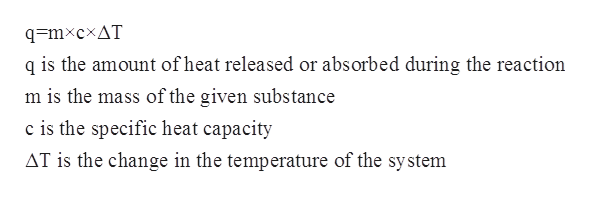# How many Cals are required to heat a 28.4 g ice cube from -23.0 °C to -1.0 °C.

Question
3 views

How many Cals are required to heat a 28.4 g ice cube from -23.0 °C to -1.0 °C.

check_circle

Step 1

The specific heat capacity for a given substance can be defined  as the amount of heat that is needed to raise the temperature of a given substance per unit of the mass. The mathematical relationship between specific heat capacity, mass, change in temperature and the heat of the solution is given by the following equation:help_outlineImage Transcriptioncloseq=mxcxAT q is the amount of heat released or absorbed during the reaction m is the mass of the given substance c is the specific heat capacity AT is the change in the temperature of the system fullscreen
Step 2

In this problem, it has been given that the mass of the ice is given to be 28.4 g.

The change in temperature is given to be from -23oC to -1oC which can be calculated as follows:

Step 3

The specific heat capacity of ice is 2.03 J/g oC.

Substituting al...

### Want to see the full answer?

See Solution

#### Want to see this answer and more?

Solutions are written by subject experts who are available 24/7. Questions are typically answered within 1 hour.*

See Solution
*Response times may vary by subject and question.
Tagged in

### Physical Chemistry